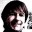# screens

### Screen words glossary

`+LOAD ( u -- )`
Add a given number `u` to the current screen number `scr` and load that screen.

`+THRU ( +from +to -- )`
Calculate the new `from` and `to` by adding the current screen number `scr` to the provided numbers `+from` and `+to`, respectively. Then, load and interpret the screen range from `from` to `to` from the current file.

`B ( -- )` "back"
Decrement the value of the screen number `scr` by 1 and display the corresponding screen.

`BY ( u <name> -- )`
Define a new word with a given name `<name>`. When `<name>` is later executed it loads screen `u`. Same as `LOADS`.

`FROM ( <filename> -- )`
Set the `fromfilename` variable to a given filename.

`HI ( -- )`

`INDEX ( from to -- )`
Print the first line of the range of screens from a given `from` to `to`, for indexing purposes.

`L ( -- )` "list"
Display the content of the current screen.

`LD ( -- )` "load"

`LIST ( u -- )`
Set the current screen to `u`, then display the current screen.

`LOAD ( u -- )`
Load and interpret screen `u` from the current file.

`LOADS ( u <name> -- )`
Define a new word with a given name `<name>`. When `<name>` is later executed it loads screen `u`. Same as `BY`.

`N ( -- )` "next"
Increment the value of the screen number `scr` by 1, then display the corresponding screen.

`QX ( -- )` "quick index"
Calculate and display the title lines of a range of 30 screens from the current screen to the 30th screen afterwards. Use for quick indexing.

`THRU ( from to -- )`
Load and interpret a screen range from a given `from` to `to` from the current file.

`USE ( <filename> -- )`
Set the `usefilename` and `fromfilename` variables to a given filename and load the corresponding file into memory.

`UTILITY ( u <name> -- )`
Define a new constant `<name>` with a given value `u`.

## by UlrichHoffmann